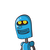# 27. If 10 power x = 64, what is the value of 10 power x/2+1?​

27. If 10 power x = 64, what is the value of 10 power x/2+1?​

### 1 thought on “27. If 10 power x = 64, what is the value of 10 power x/2+1?​”

1.Step-by-step explanation:

10ˣ = 64  ⇔  log₁₀64 = x

log₁₀8² = x  ⇒  2×log₁₀8 = x  ⇒  log₁₀8 = x/2 ..1

To find 10^(x/2)+1

substitute x/2 = log₁₀8

10^( log₁₀8 + 1) = y

10^(log₁₀8 + log₁₀10) = y (note : logₓx = 1 and logₓa + logₓb = logₓab)

10^(log₁₀80) = y

log₁₀10^(log₁₀80) = log₁₀y

(log₁₀80)×(log₁₀10) = log₁₀y (note : logₓ(a^logₙb) = (logₙb) × ( logₓa) )

log₁₀80 × 1 = log₁₀y

80 = y

y = 10^((x/2)+1) = 80

Hope it helps. Mark as brainliest if it was helpful. Thank you.